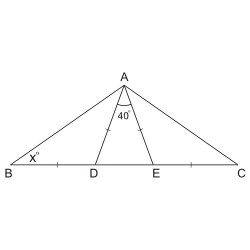We cover every section of the GMAT with in-depth lessons, 5000+ practice questions and realistic practice tests.

## Up to 90+ points GMAT score improvement guarantee

### The best guarantee you’ll find

Our Premium and Ultimate plans guarantee up to 90+ points score increase or your money back.

## Master each section of the test

### Comprehensive GMAT prep

We cover every section of the GMAT with in-depth lessons, 5000+ practice questions and realistic practice tests.

## Schedule-free studying

### Learn on the go

Study whenever and wherever you want with our iOS and Android mobile apps.

# Triangles: IsoscelesIf $$BD=DA = EA = CE$$ as shown above, what is the value of $$x$$?
Correct. [[snippet]] Lines $$AD$$ and $$AE$$ are equal so angles $$ADE$$ and $$AED$$ are equal, and > $$\displaystyle \angle ADE = \angle AED = \frac{180º-40º}{2} = 70º$$. Since $$BDA$$ is a supplementary angle of angle $$ADE$$, angle $$BDA = 180º - 70º = 110º$$. Based on this, > $$\displaystyle{xº = \frac{180º - 110º}{2} = 35º}$$.
Incorrect. [[snippet]]
Incorrect. [[snippet]]
Incorrect. [[snippet]]
Incorrect. [[snippet]]
15
20
25
30
35